# Dice Problem¶

Suppose you have a singular die and you roll it. Then you roll the number of dice that show on the last roll you made. Repeat this until you have rolled at least 100.

What numbers do you expect not to roll during the last round?

In :
import random as r
from pylab import *
from numba import njit

In :
@njit
def rolls():
numDice = 1
while numDice < 100:
newNumDice = 0
for i in range(numDice):
newNumDice += r.randint(1,6)
numDice = newNumDice
return numDice

In :
def run(trials):
numDiceDict = {}
for _ in range(trials):
numDice = rolls()
if numDice in numDiceDict:
numDiceDict[numDice] += 1/trials
else:
numDiceDict[numDice] = 1/trials
print("number rolled on the last round vs. frequency")
bar(list(numDiceDict.keys()), list(numDiceDict.values()));

In :
run(10000)

number rolled on the last round vs. frequency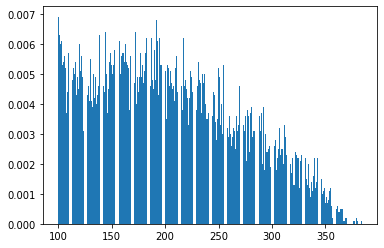In :
run(10000)

number rolled on the last round vs. frequency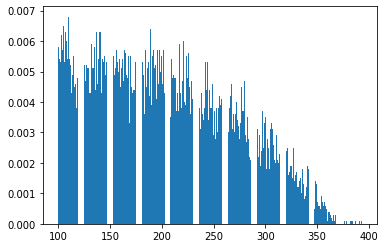In :
run(10000)

number rolled on the last round vs. frequency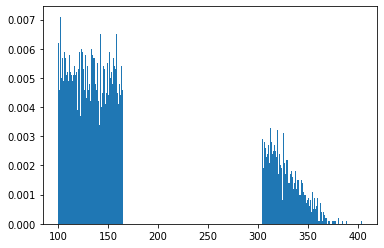In :
run(10000)

number rolled on the last round vs. frequency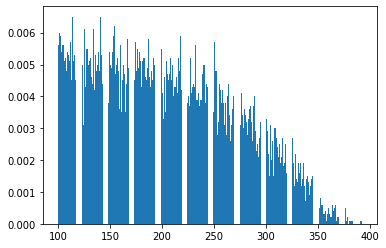In :
run(10000)

number rolled on the last round vs. frequency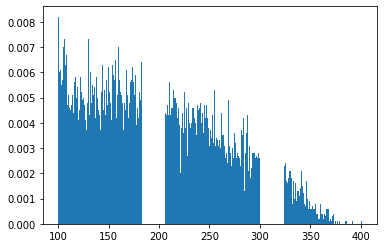In :
run(10000)

number rolled on the last round vs. frequency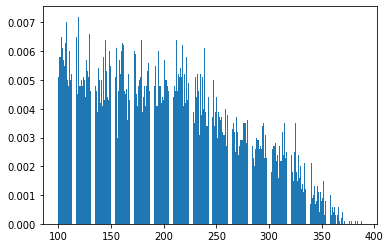In :
run(10000)

number rolled on the last round vs. frequency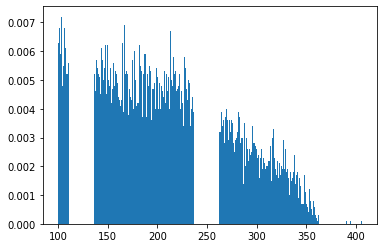# Why are the bands different?!¶

In [ ]: